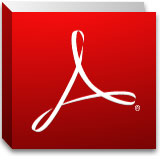You are hereCourses / Mathematics / Algebra I Common Core S1 (#413)

# Algebra I Common Core S1 (#413)

Course Description:

This course provides instruction on the essential fundamentals of algebra such as relationships between quantities, as expressed by written expressions, formulas, equations, graphs, functions, fundamental number operations with the different sets of numbers, fractions and problem solving. Students will use the order of operations to simplify expressions, evaluate expressions, and solve equations and inequalities, use line graphs and the Cartesian plane to represent solutions. Students will also be introduced to linear functions and exponential functions as well as systems of equations and inequalities.

This course is based on the book “Common Core Algebra I”. It is published by Pearson, 2011. ISBN: 9780133185492. However, you will not need to order a book. When you register for the course, you will have access to the online version of the textbook.

## Notice to Prospective StudentsStudents must have a valid e-mail address, physical mailing address and access to any free PDF file viewer, like Adobe Reader, for filling out course materials online and printing course materials at home.